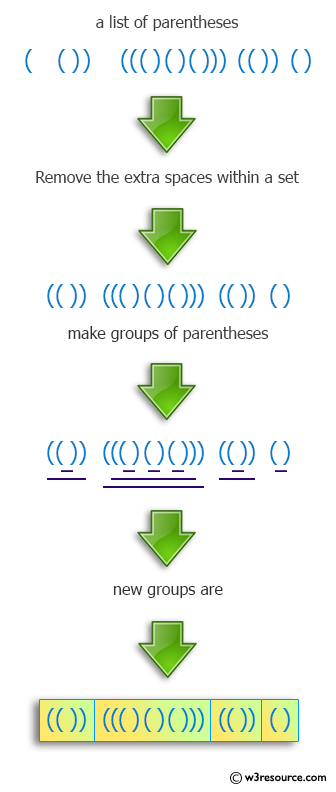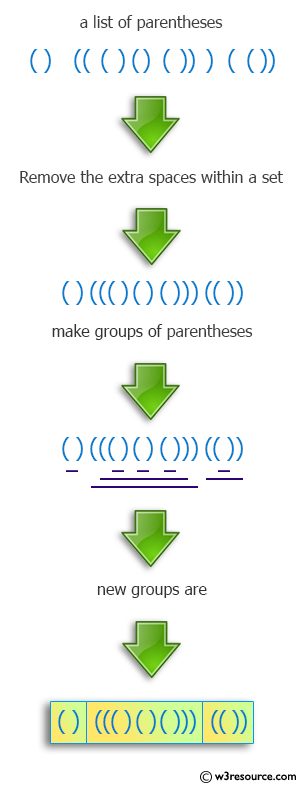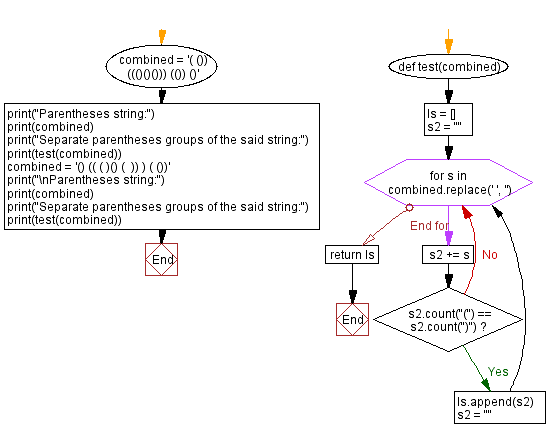﻿ Python: Separate Parentheses Groups Perfectly - w3resource# Python: Separate Parentheses Groups Perfectly

## Python Programming Puzzles: Exercise-10 with Solution

Given a string consisting of whitespace and groups of matched parentheses, write a Python program to split it into groups of perfectly matched parentheses without any whitespace.

```Input:
( ()) ((()()())) (()) ()
Output:
['(())', '((()()()))', '(())', '()']

Input:
() (( ( )() (  )) ) ( ())
Output:
['()', '((()()()))', '(())']
```

Pictorial Presentation:Sample Solution:

Python Code:

``````#License: https://bit.ly/3oLErEI

def test(combined):
ls = []
s2 = ""
for s in combined.replace(' ', ''):
s2 += s
if s2.count("(") == s2.count(")"):
ls.append(s2)
s2 = ""
return ls
combined = '( ()) ((()()())) (()) ()'
print("Parentheses string:")
print(combined)
print("Separate parentheses groups of the said string:")
print(test(combined))
combined = '() (( ( )() (  )) ) ( ())'
print("\nParentheses string:")
print(combined)
print("Separate parentheses groups of the said string:")
print(test(combined))
``````

Sample Output:

```Parentheses string:
( ()) ((()()())) (()) ()
Separate parentheses groups of the said string:
['(())', '((()()()))', '(())', '()']

Parentheses string:
() (( ( )() (  )) ) ( ())
Separate parentheses groups of the said string:
['()', '((()()()))', '(())']
```

Flowchart:## Visualize Python code execution:

The following tool visualize what the computer is doing step-by-step as it executes the said program:

Python Code Editor :

Have another way to solve this solution? Contribute your code (and comments) through Disqus.

What is the difficulty level of this exercise?

Test your Programming skills with w3resource's quiz.

﻿

## Python: Tips of the Day

Clamps num within the inclusive range specified by the boundary values x and y:

Example:

```def tips_clamp_num(num,x,y):
return max(min(num, max(x, y)), min(x, y))
print(tips_clamp_num(2, 4, 6))
print(tips_clamp_num(1, -1, -6))
```

Output:

```4
-1
```